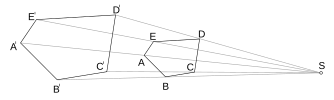# Dilation (geometry) facts for kids

Kids Encyclopedia FactsTwo similar geometric figures related by a dilation, with the center of dilation at S.

In geometry, dilation (also called homothety or homothetic transformation) is the process which changes the size of a figure without changing its shape, a type of transformation. Any figure and its image after dilation are similar to each other.

Dilation is defined by a point V, the center of dilation, and a number k, the ratio. Given a point P in the plane, its image P' (P prime) is on the line VP. The distance from P' to V is k times the distance from P to V.Dilation (geometry) Facts for Kids. Kiddle Encyclopedia.